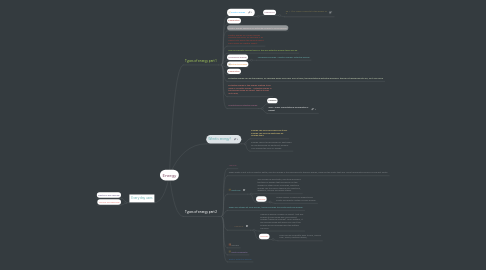# EnergyPaola MACIEL
Get Started. It's FreeEnergy## 2. Types of energy part 1

### 2.1. Kinetic energy

2.1.1. FORMULA

2.1.1.1. KE = 1/2 x Mass x Velocity to the power of 2

### 2.6. Mechanical energy

2.6.1. Mechanical energy = kinetic energy+Potential energy

### 2.11. Gravitational potential energy

2.11.1. Formula

2.11.2. GPE = mass x gravitational accelaration x height

## 4. Types of energy part 2

### 4.3. Electrical

4.3.1. Also known as electricity, electrical energy is the type of energy that influences on the change of other forms of energy, electrical energy can transform thermal into electrical, chemical, nuclear and many others.

4.3.2. Sources

4.3.2.1. Mainly fossils. Fossils are prehistorical plants and plants contain nuclear energy.

### 4.5. Chemical

4.5.1. Chemical energy is when an object that has energy stored inside has a permanent change (chemical change). Like a battery, it has energy inside but when you use it the energy will be absorbed and the battery can burn.

4.5.2. Sources

4.5.2.1. The sources are pretty easy to find, There's Coal, Wood, Photosynthesis,